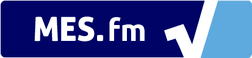We notice that you are using AdBlock. Please whitelist Mortgage Calculator to help keep this site up and running! :)# Mortgage Amortization Formula with Balloon Payment

A = \frac{iP(1+i)^n}{(1+i)^n - 1} - \frac{iB}{(1+i)^{n+1} - (1+i)}

where:

• A = periodic payment amount
• P = amount of principle or loan owing (subtracting any down-payments)
• i = period interest rate
• n = total number of payments
• B = Final Balloon Payment
• If B = 0, then the amortization formula is the same as the basic one

You can watch the proof of this formula here: